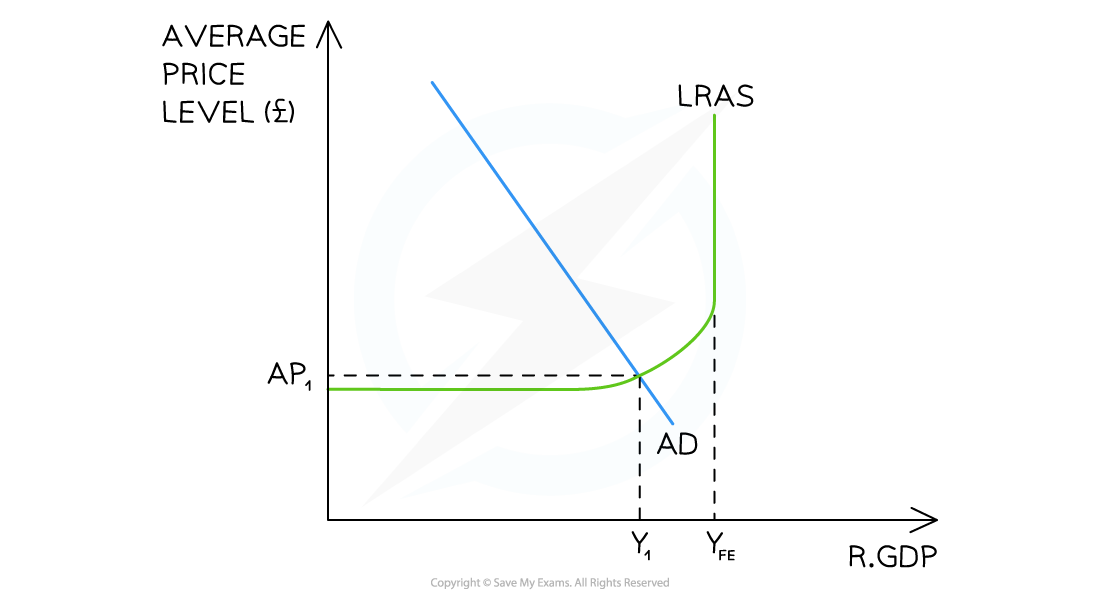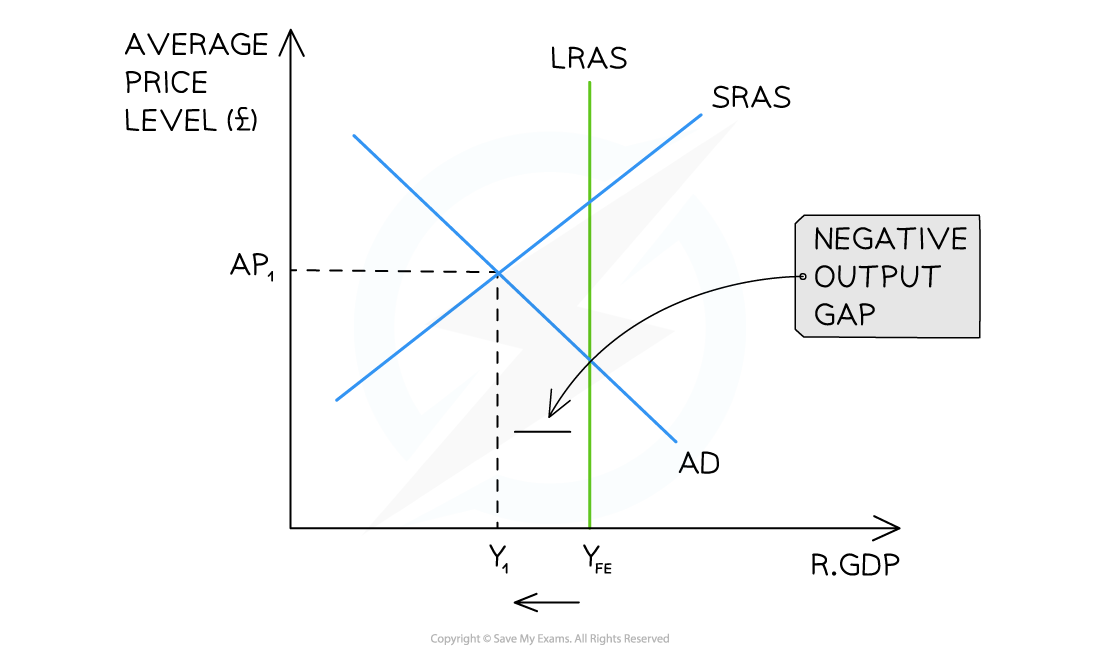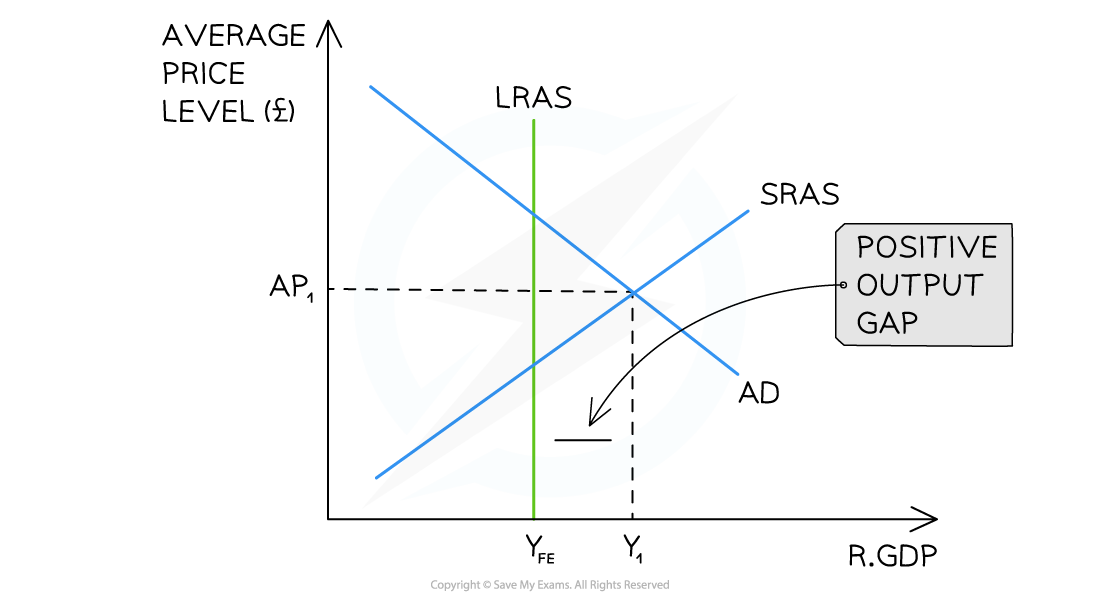# Edexcel A Level Economics A:复习笔记2.5.2 Output Gaps

### Actual Growth & Long-term Growth

• Actual growth can be differentiated from the idea of long-term trends in growth rates
• A long-term growth trend is the underlying trend rate of economic growth over a longer period of time
• This is determined by the constant increases in the productive capacity of an economy (aggregate supply)
• The increase in productive capacity is illustrated by a rightward shift of the long-run aggregate supply curve (LRAS)
• Use of long-term growth trends can reduce the impact of outliers in the data

### Positive & Negative Output Gaps

• An output gap is the difference between the actual level of output (real GDP) and the maximum potential level of output
• A positive output gap occurs when real GDP is greater than the potential real GDP
• A negative output gap occurs when the real GDP is less than the potential real GDP
• There is spare capacity in the economy to produce more goods/services than are being produced
• It is difficult to measure output gaps accurately
• This is because it is hard to know exactly what the maximum productive potential of an economy is
• Rapidly rising prices can indicate a positive gap is developing
• Rising unemployment and slowdown in economic growth can indicate that a negative gap is increasing

#### A Negative Output GapAn Keynesian (top) and Classical (bottom) diagram illustrating an economy that has a negative output gap (Y1- YFE) and is currently producing less than its potential output

Diagram Analysis

• The potential output of this economy is at YFE
• The economy is in a short-run equilibrium at AP1Y1
• A negative output gap exists at Y1 - YFE
• This effectively gives the economy sparer capacity in the short-term
• One cause of this may be that the AD has recently decreased due to a fall in consumption
• The Classical view is that the output will return to YFE  in the long-run, but at a lower average price level
• The Keynesian view is that an economy may be stuck in a negative output gap for a long period of time

#### A Positive Output GapAn AD/AS diagram illustrating an economy that has a positive output gap (YFE - Y1) and is currently producing more than its potential output

Diagram Analysis

• The potential output of this economy is at YFE
• The economy is in a short-run equilibrium at AP1Y1
• A positive output gap exists at YFE - Y1
• This effectively gives the economy more productive capacity in the short-term
• One cause of this may be that workers are willing to work overtime once full capacity is reached
• It is not sustainable and the Classical view is that the output will return to YFE, but at a higher price level